Open in App
Not now

# Latches in Digital Logic

• Difficulty Level : Medium
• Last Updated : 21 Jun, 2022

Latches are basic storage elements that operate with signal levels (rather than signal transitions). Latches controlled by a clock transition are flip-flops. Latches are level-sensitive devices. Latches are useful for the design of the asynchronous sequential circuit. Latches are sequential circuit with two stable states. These are sensitive to the input voltage applied and does not depend on the clock pulse. Flip flops that do not use clock pulse are referred to as latch.

SR (Set-Reset) Latch – They are also known as preset and clear states. The SR latch forms the basic building blocks of all other types of flip-flops.

SR Latch is a circuit with:
(i) 2 cross-coupled NOR gate or 2 cross-coupled NAND gate.
(ii) 2 input S for SET and R for RESET.
(iii) 2 output Q, Q’.

Under normal conditions, both the input remains 0. The following is the RS Latch with NAND gates: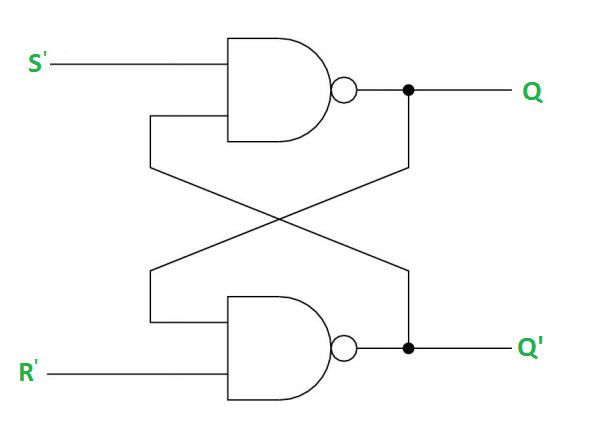Case-1: S’=R’=1 (S=R=0) –
If Q = 1, Q and R’ inputs for 2nd NAND gate are both 1.
If Q = 0, Q and R’ inputs for 2nd NAND gate are 0 and 1 respectively.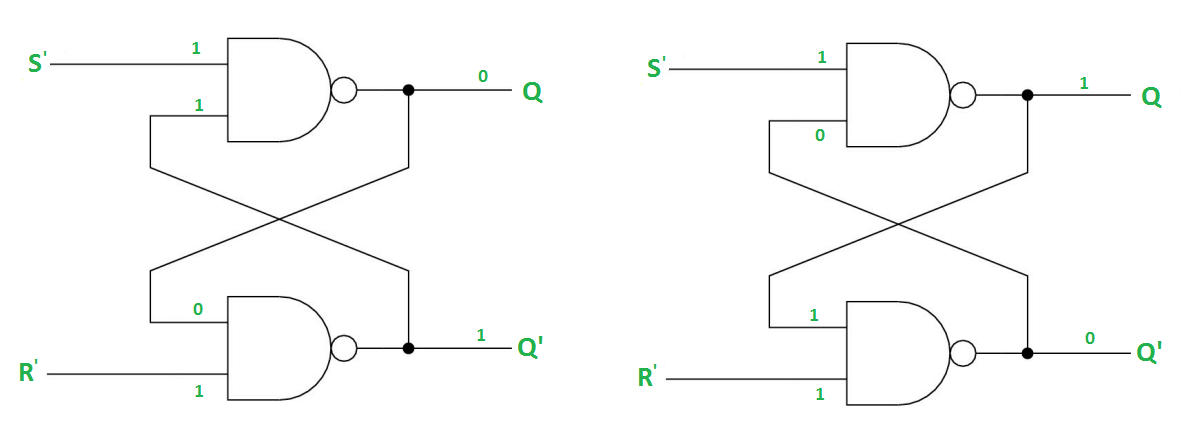Case-2: S’=0, R’=1 (S=1, R=0) –
As S’=0, the output of 1st NAND gate, Q = 1(SET state). In 2nd NAND gate, as Q and R’ inputs are 1, Q’=0.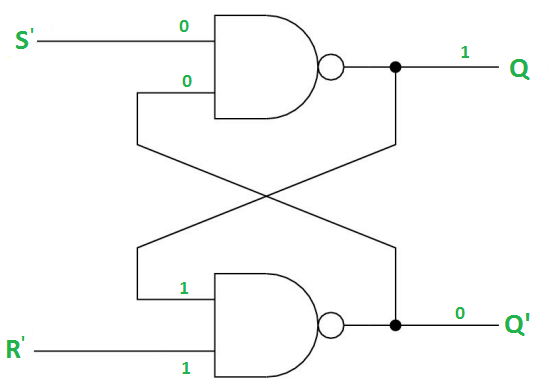Case-3: S’= 1, R’= 0 (S=0, R=1) –
As R’=0, the output of 2nd NAND gate, Q’ = 1. In 1st NAND gate, as Q and S’ inputs are 1, Q=0(RESET state).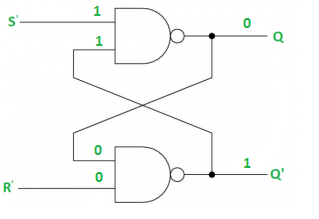Case-4: S’= R’= 0 (S=R=1) –
When S=R=1, both Q and Q’ becomes 1 which is not allowed. So, the input condition is prohibited.

The SR Latch using NOR gate is shown below: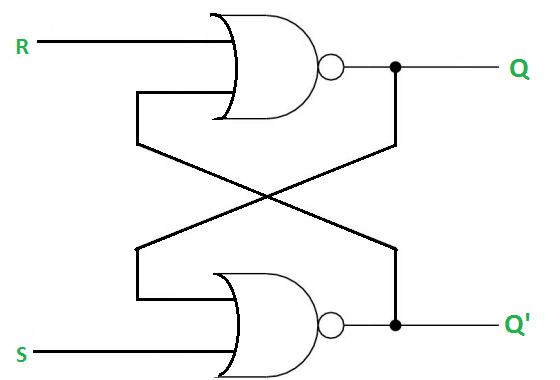Gated SR Latch –
A Gated SR latch is a SR latch with enable input which works when enable is 1 and retain the previous state when enable is 0.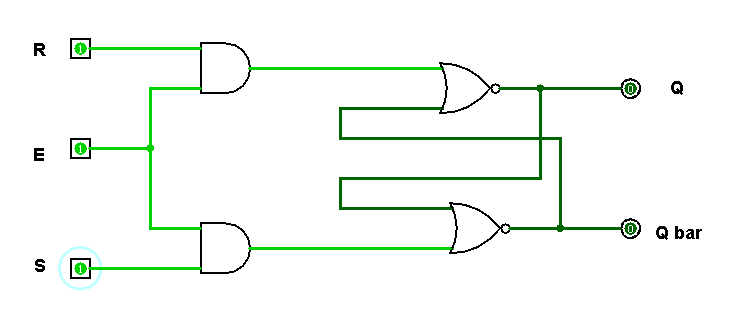Gated D Latch –
D latch is similar to SR latch with some modifications made. Here, the inputs are complements of each other. The letter  in the D latch stands for “data” as this latch stores single bit temporarily.

The design of D latch with Enable signal is given below: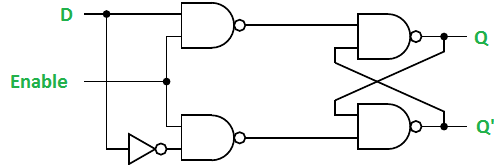The truth table for the D-Latch is shown below:

As the output is same as the input D, D latch is also called as Transparent Latch. Considering the truth table, the characteristic equation for D latch with enable input can be given as:

`Q(n+1) = EN.D + EN'.Q(n)`

Reference:
DIGITAL ELECTRONICS – Atul P. Godse, Mrs. Deepali A. Godse

My Personal Notes arrow_drop_up